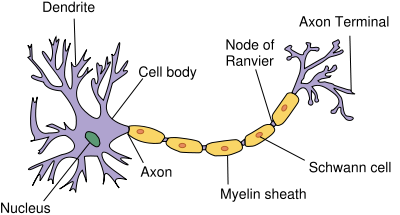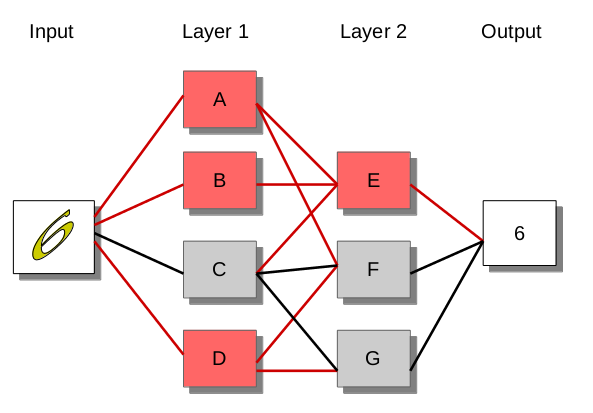# Deep Learning

Python hosting: Host, run, and code Python in the cloud!

Deep learning is the new big trend in Machine Learning. It is about artificial neural networks (ANN for short) that consists of many layers.

While artificial neural networks have existed for over 40 years, the Machine Learning field had a big boost partly due to hardware improvements.

Related course: Practical Deep Learning: A Python-Based Introduction

Neural networks
In the field of Machine Learning, artificial neural networks (ANN) are computational models that are inspired by the field of biology: biological neural networks.

The (human) brain has a network of neurons, approximately 86 billion neurons (estimated number). Neurons are used to transfer information from A to B. During this process it passes neurons which are activated by the brain.

A neuron receives input signals from other signals using its receivers (called ‘dendrites’). The neuron has a threshold, if the amount of signals are above its treshold it will send an electric pulse to the dendrites of other neurons (the output of a neuron is called ‘terminals’).Neuron, source: wikimedia

Artificial Neural Networks
An artificial neural network (ANN) is a mathematical model that is modeled after biological neural networks. Just like biological neural networks have dendrites (input) and terminals (outputs), every ANN has an input node and an output node. Every ANN has connected nodes which are setup in layers. The individual nodes have inputs and outputs, similar to the biological neuron.Artificial neural network, 2 layer

Consider the task of digital character recognition. The input is an image containing a character. The output is the character that is recognized. Neurons in the first layer are activated once an input image is given, starting a chain reaction. In the image below you see several of the neurons are activated. Each neuron acts according to rules (training data). If a node is not activated it remains gray. The output is based on the layer of neurons directly before it.

Related course: Practical Deep Learning: A Python-Based Introduction

Why is it called ‘Deep Learning’?
When Artificial neural networks were first created, roughly 40 years ago, the neural networks were only 2 layers deep. During this period there had been computational limitations preventing the scientists from adding more layers. These days it is common to have neural networks that have 10+ or 100+ layers.